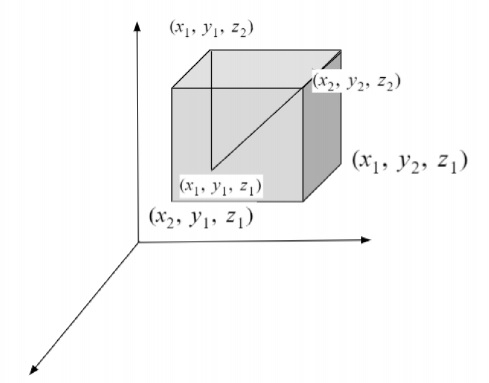# C program to calculate distance between three points in 3D

Given with the 3-D plane and hence three coordinates and the task is to find the distance between the given points and display the result.

In a three dimension plane there are three axis that are x-axis with its coordinates as (x1, y1, z1), y-axis with its coordinates as (x2, y2, z2) and z-axis with its coordinates as (x3, y3, z). To calculate the distance between them there is a direct formula which is given below

$$\sqrt{\lgroup x2-x1\rgroup^{2}+\lgroup y2-y1\rgroup^{2}+\lgroup z2-z1\rgroup^{2}}$$

Given below is the diagram representing three different axis and their coordinatesApproach used below is as follows

• Input the coordinates as (x1, y1, z1), (x2, y2, z2) and (x3, y3, z3)
• Apply the formula to compute the difference between these points
• Print the distance

## Algorithm

Start
Step 1-> declare function to calculate distance between three point
void three_dis(float x1, float y1, float z1, float x2, float y2, float z2)
set float dis = sqrt(pow(x2 - x1, 2) + pow(y2 - y1, 2) + pow(z2 - z1, 2) * 1.0)
print dis
step 2-> In main()
Set float x1 = 4
Set float y1 = 9
Set float z1 = -3
Set float x2 = 5
Set float y2 = 10
Set float z2 = 9
Call three_dis(x1, y1, z1, x2, y2, z2)
Stop

## Example

#include <stdio.h>
#include<math.h>
//function to find distance bewteen 3 point
void three_dis(float x1, float y1, float z1, float x2, float y2, float z2) {
float dis = sqrt(pow(x2 - x1, 2) + pow(y2 - y1, 2) + pow(z2 - z1, 2) * 1.0);
printf("Distance between 3 points are : %f", dis);
return;
}
int main() {
float x1 = 4;
float y1 = 9;
float z1 = -3;
float x2 = 5;
float y2 = 10;
float z2 = 9;
three_dis(x1, y1, z1, x2, y2, z2);
return 0;
}

## Output

IF WE RUN THE ABOVE CODE IT WILL GENERATE FOLLOWING OUTPUT

Distance between 3 points are : 12.083046